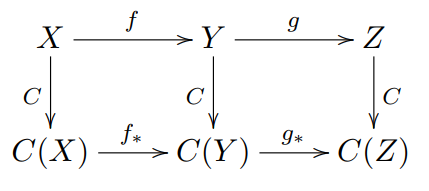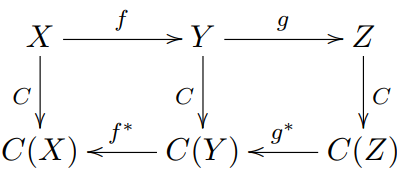## 范畴

### 范畴的概念

• 一个由一些 对象 (objects) 所构成的类 $\mathrm{ob} (\mathcal{C})$

• 对象间的 态射(morphism) 所构成的类 $\mathrm{Hom} (\mathcal{C})$

态射是指从一个“源物件”对象 $a$ 到“目标对象” $b$ 的映射 $f:a\mapsto b$，其中 $a,b\in\mathrm{ob} (\mathcal{C})$。所有从 $a$$b$ 的态射组成的类称为 态射类，记为 $\mathrm{Hom} (a,b)$

• 对任三个对象 $a,b,c$，有二元运算：

$\mathrm{Hom} (a,b)\times\mathrm{Hom} (b,c)\rightarrow \mathrm{Hom} (a,c)$

• 结合律
$f \in \mathrm{Hom} (a,b), g \in \mathrm{Hom} (b,c),h \in \mathrm{Hom} (c,d)$，则：

$h\circ (g\circ f) = (h\circ g)\circ f$

• 存在 恒等态射
对任意一个对象 $a$$\mathrm{Hom} (a,a)$ 中存在唯一元素，记为 $\mathrm{Id}_a$，使得:

\begin{aligned} &f\circ \mathrm{Id}_a = f,\ \forall f \in \mathrm{Hom} (a,b)\\ &\mathrm{Id}_a \circ g = g,\ \forall g \in \mathrm{Hom} (b,a)\\ \end{aligned}

$f\circ g = \mathrm{Id}_b,\ g\circ f = \mathrm{Id}_a .$

### 函子

$(\mathscr{A},\mathrm{Mor}(\mathscr{A}))$$(\mathscr{B},\mathrm{Mor}(\mathscr{B}))$ 为范畴，从 $(\mathscr{A},\mathrm{Mor}(\mathscr{A}))$$(\mathscr{B},\mathrm{Mor}(\mathscr{B}))$ 的函子是一个映射 $C$

$C: (\mathscr{A},\mathrm{Mor}(\mathscr{A})) \rightarrow (\mathscr{B},\mathrm{Mor}(\mathscr{B}))$

$C$ 是一个范畴间的映射。

• $f: X \rightarrow Y$ 是一个态射，那么 $C(f)$ 为如下态射：

$C(f):C(X)\rightarrow C(Y)$

• $f,g\in\mathrm{Mor}(\mathscr{A})$，且它们可以复合，则有：

$C(f)\circ C(g) = C(f\circ g)$

• 保持恒元：

$C(\mathrm{Id}_X) = \mathrm{Id}_{C(X)}$• $f,g\in\mathrm{Mor}(\mathscr{A})$，且它们可以复合，则有：

$C(f)\circ C(g) = C(g\circ f)$## 关系

### 等价关系

• 自反性：$(x,x) \in R,\ \forall x \in X$
• 对称性：$(x,y) \in R \Rightarrow (y,x) \in R,\ \forall x,y \in X$
• 传递性：$(x,y) \in R\ and \ (y,z) \in R ⇒ (x,z) \in R,\ \forall x,y,z \in X$

$x$$y$ 之间存在等价关系，记为 $x\sim y$

$x$ 所在的等价类，记为 $[x]$。它是 $X$ 的一个子集，定义为:

$[x] \equiv \{y|y ∼ x\}.$

### 偏序关系

• 自反性：$(x,x) \in R,\ \forall x \in X$
• 反对称性：$(x,y) \in R\ and \ (y,x) \in R \Rightarrow x = y, \ \forall x,y \in X$
• 传递性：$(x,y) \in R \ and\ (y,z)\in R\Rightarrow (x,z)\in R,\ \forall x,y,z\in R$

$x$$y$ 由偏序 $R$ 相关，我们可以将其记为 $x \preccurlyeq y$